## Atomic Structure Questions and Answers Part-17

1.The ionization enthalpy of hydrogen atom is $1.312 ×10^{6}J mol^{-1}$    . The energy required to excite the electron in the atom from n = 1 to n = 2 is
a) $9.84 ×10^{5}J mol^{-1}$
b) $6.56 ×10^{5}J mol^{-1}$
c) $7.56 ×10^{5}J mol^{-1}$
d) $8.51 ×10^{5}J mol^{-1}$

Explanation: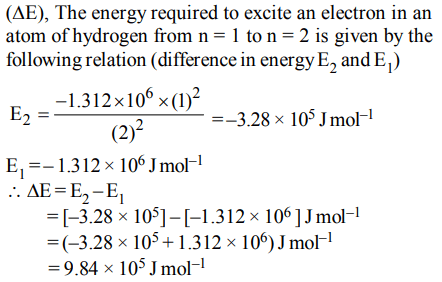2. Which one of the following constitutes a group of the isoelectronic species?
a) $C_2^{2-},O_2^-,CO,NO$
b) $CN^{-},N_{2},O_2^{2-},C_2^{2-},$
c) $NO^{+},C_2^{2-},CN^{-},N_{2}$
d) $N_{2},O_2^-,NO^{+},CO$

Explanation: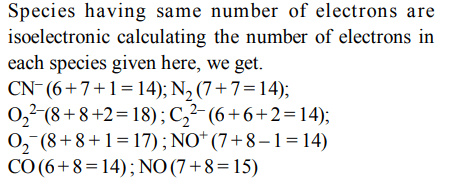3. Calculate the wavelength (in nanometer) associated with a proton moving at $1.0 ×10^{3}ms^{-1}$ .   (Mass of proton = $1.67 ×10^{-27}$     Kg and h = $6.63 × 10^{-34}$     Js)
a) 0.40 nm
b) 2.5 nm
c) 14.0 nm
d) 0.32 nm

Explanation:4. In an atom, an electron is moving with a speed of 600 m/s withan accuracy of 0.005%. Certainity with which the position of the electron can be located is $\left(h = 6.6 × 10^{-34}Kg m^{2}s^{-1},mass of electron,e_{m}=9.1 × 10^{-31}Kg\right)$
a) $5.10 × 10^{-3}m$
b) $1.92 × 10^{-3}m$
c) $3.84 × 10^{-3}m$
d) $1.52 × 10^{-4}m$

Explanation: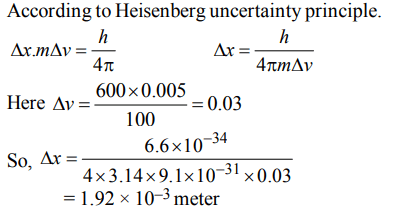5. The energy required to break one mole of Cl – Cl bonds in$CI_{2}$ is $242 kJMol^{-1}$  .The longest wavelength of light capable of breaking a single Cl – Cl bond is $\left(c = 3 ×10^{8}ms^{-1} andN_{A}=6.02 ×10^{23}mol^{-1}\right)$
a) 594 nm
b) 640 nm
c) 700 nm
d) 494 nm

Explanation: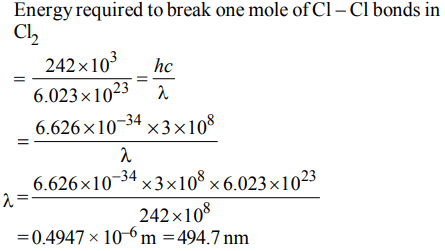6. Ionisation energy of $He^{+}$ is $19.6 × 10^{-18}J atom^{-1}$    . The energy of the first stationary state $(n = 1) of Li^{2+}$    is
a) $4.41 × 10^{-16} J atom^{-1}$
b) $–4.41 × 10^{-17} J atom^{-1}$
c) $–2.2 × 10^{-15} J atom^{-1}$
d) $8.82 × 10^{-17} J atom^{-1}$

Explanation: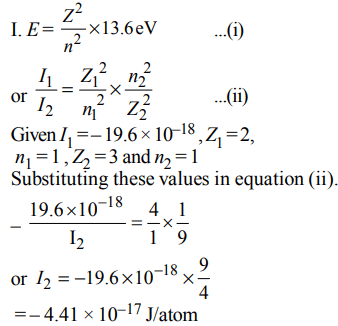7. The frequency of light emitted for the transition n = 4 to n = 2 of the $He^{+}$ is equal to the transition in H atom corresponding to which of the following ?
a) n = 2 to n = 1
b) n = 3 to n = 2
c) n = 4 to n = 3
d) n = 3 to n = 1

Explanation: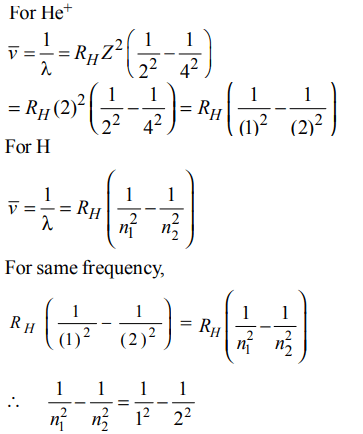n1 = 1 & n2 = 2

8. The electrons identified by quantum numbers $n$ and $\ell$ :
(A) $n = 4,\ell=1$
(B) $n = 4,\ell=0$
(C) $n = 3,\ell=2$
(D) $n = 3,\ell=1$
can be placed in order of increasing energy as :
a) (C) < (D) < (B) < (A)
b) (D) < (B) < (C) < (A)
c) (B) < (D) < (A) < (C)
d) (A) < (C) < (B) < (D)

Explanation:
(A) 4 p
(B) 4 s
(C) 3 d
(D) 3 p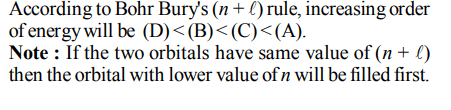9.The increasing order of the ionic radii of the given isoelectronic species is
a) $Cl^{-},Ca^{2+},K^{+},S^{2-}$
b) $S^{2-},Cl^{-},Ca^{2+},K^{+}$
c) $Ca^{2+},K^{+},Cl^{-},S^{2-}$
d) $K^{+},S^{2-},Ca^{2+},Cl^{-}$10. Energy of an electron is given by $E=– 2.178 ×10^{-18}J\left[\frac{Z^{2}}{n^{2}}\right]$     Wavelength of light required to excite an electron in an hydrogen atom from level n = 1 to n = 2 will be :$\left(h=6.62 × 10^{-34}Js, c = 3.0 ×10^{8}ms^{-1}\right)$
a) $1.214 × 10^{-7}m$
b) $2.816 × 10^{-7}m$
c) $6.500 × 10^{-7}m$
d) $8.500 × 10^{-7}m$